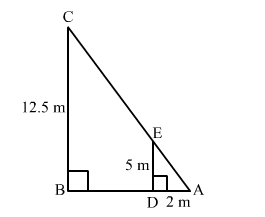# The shadow of a 5-m-long stick is 2 m long. At the same time the length of the shadow of a 12.5 mQuestion:

The shadow of a 5-m-long stick is 2 m long. At the same time the length of the shadow of a 12.5 m high tree (in m) is
(a) 3.0
(b) 3.5
(c) 4.5
(d) 5.0

Solution:Suppose DE is a 5 m long stick and BC is a 12.5 m high tree.
Suppsose DA and BA are the shadows of DE and BC respectively.
∠A = ∠A             (Common)
By AA-similarity criterion

If two triangles are similar, then the the ratio of their corresponding sides are equal.

$\therefore \frac{\mathrm{AB}}{\mathrm{AD}}=\frac{\mathrm{BC}}{\mathrm{DE}}$

$\Rightarrow \frac{\mathrm{AB}}{2}=\frac{12.5}{5}$

$\Rightarrow \mathrm{AB}=5 \mathrm{~cm}$

Hence, the correct answer is option (d).